Gram-Schmidt Orthogonalization Next  |  Prev  |  Up  |  Top  |  Index  |  JOS Index  |  JOS Pubs  |  JOS Home  |  Search

### Gram-Schmidt Orthogonalization

Recall from the end of §5.10 above that an orthonormal set of vectors is a set of unit-length vectors that are mutually orthogonal. In other words, orthonormal vector set is just an orthogonal vector set in which each vector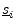has been normalized to unit length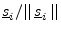.

Theorem: Given a set of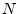linearly independent vectors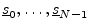from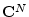, we can construct an orthonormal set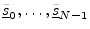which are linear combinations of the original set and which span the same space.

Proof: We prove the theorem by constructing the desired orthonormal set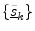sequentially from the original set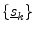. This procedure is known as Gram-Schmidt orthogonalization.

First, note that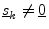for all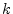, since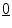is linearly dependent on every vector. Therefore,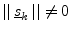.

• Set.
• Define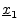as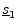minus the projection ofonto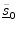: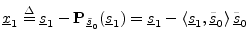The vectoris orthogonal toby construction. (We subtracted out the part ofthat wasn't orthogonal to.) Also, sinceand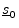are linearly independent, we have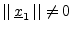.

• Set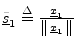(i.e., normalize the result of the preceding step).
• Define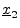as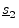minus the projection ofontoand:• Normalize: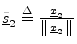.
• Continue this process until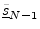has been defined.

The Gram-Schmidt orthogonalization procedure will construct an orthonormal basis from any set oflinearly independent vectors. Obviously, by skipping the normalization step, we could also form simply an orthogonal basis. The key ingredient of this procedure is that each new basis vector is obtained by subtracting out the projection of the next linearly independent vector onto the vectors accepted so far into the set. We may say that each new linearly independent vector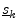is projected onto the subspace spanned by the vectors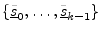, and any nonzero projection in that subspace is subtracted out ofto make the new vector orthogonal to the entire subspace. In other words, we retain only that portion of each new vectorwhich points along'' a new dimension. The first direction is arbitrary and is determined by whatever vector we choose first (here). The next vector is forced to be orthogonal to the first. The second is forced to be orthogonal to the first two (and thus to the 2D subspace spanned by them), and so on.

This chapter can be considered an introduction to some important concepts of linear algebra. The student is invited to pursue further reading in any textbook on linear algebra, such as .5.13

Matlab/Octave examples related to this chapter appear in Appendix I.

Next  |  Prev  |  Up  |  Top  |  Index  |  JOS Index  |  JOS Pubs  |  JOS Home  |  Search

[How to cite this work] [Order a printed hardcopy]